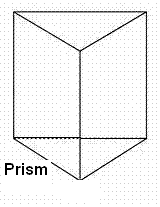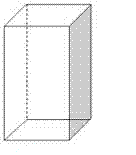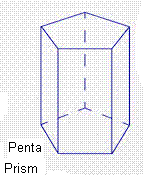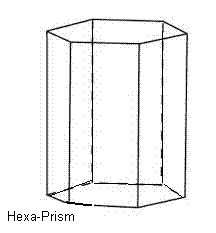# Prism Calculator

Calculate the various properties of Triangular Prism, Rectangular Prism(Cuboid), Regular Square Prism(Square Cuboid), Regular Pentagonal Prism, Regular Hexagonal Prism, for given values.

### Triangular Prism

 Enter the Altitude Enter the Base Enter the Height
 Enter the Sides

 Area of Base: Perimeter of Base: Surface Area of Prism: Volume of Prism:Triangular Prism
Area of Base :[ ½ab ]
Perimeter of Base :[ s1 + s2 + s3 ]
Surface Area of Prism :[ ab + (s1 + s2 + s3)h ]
Volume of Prism :[ ½abh ]

### Rectangular Prism

 Enter the Length Enter the Width Enter the Height

 Area of Base: Perimeter of Base: Surface Area of Prism(Cuboid): Volume of Prism(Cuboid): Diagonal of Prism(Cuboid):Rectangular Prism(Cuboid)
Area of Base :[ lw ]
Perimeter of Base :[ 2l + 2w ]
Surface Area of Prism(Cuboid) :[ 2(lw) + (2l + 2w)h ]
Volume of Prism(Cuboid) :[ lwh ]
Diagonal of Prism(Cuboid) :[ Sqrt( l² + w² + h² ) ]

### Regular Square Prism

 Enter the Side Enter the Height

 Area of Base: Perimeter of Base: Surface Area of Prism(Square Cuboid): Volume of Prism(Square Cuboid):Regular Square Prism(Square Cuboid)
Area of Base :[ ]
Perimeter of Base :[ 4s ]
Surface Area of Prism(Square Cuboid) :[ 2s² + 4sh ]
Volume of Prism(Square Cuboid) :[ s²h ]

### Regular pentagonal Prism

 Enter the Apothem Length Enter the Side Length Enter the Height

 Area of Base: Perimeter of Base: Surface Area of Prism: Volume of Prism:Regular Pentagonal Prism
Area of Base :[ ½×a×5×s ]
Perimeter of Base :[ 5s ]
Surface Area of Prism :[ 5as + 5sh ]
Volume of Prism :[ (5/2)ash ]

### Regular hexagonal Prism

 Enter the Apothem Length Enter the Side Length Enter the Height

 Area of Base: Perimeter of Base: Surface Area of Prism: Volume of Prism:Regular Hexagonal Prism
Area of Base :[ ½×a×6×s ]
Perimeter of Base :[ 6s ]
Surface Area of Prism :[ 6as + 6sh ]
Volume of Prism :[ 3ash ]

 Prism Type Definition Shape Triangular Prism The Prism having triangular bases.Rectangular Prism(Cuboid) The Prism having rectangular bases.Regular Square Prism(Square Cuboid) The Prism having square bases.Regular Pentagonal Prism The Prism having pentagonal bases.Regular Hexagonal Prism The Prism having hexagonal bases.